﻿ 基于LMDI方法对河北省终端能源消费影响因素分解分析

# 基于LMDI方法对河北省终端能源消费影响因素分解分析Factor Decomposition Analysis of Terminal Energy Consumption in Hebei Province Based on LMDI

Abstract: Energy consumption issue is getting more and more attention. This article, under the background of “the 11th Five-Year” and “the 12th Five-Year” development in Hebei Province, analyzes the changes of terminal energy consumption for each industrial sector and energy structure by using the logarithmic mean Divisia index method in Hebei province from 2005 to 2015, finds the influencing factors and gives explanations. We can conclude that the expansion of economic production scale is the decisive factor to promote the growth of energy consumption in Hebei Province, and energy technology factor is the effective measure to reduce energy consumption. The influence of energy structure effect is very small and the influence of industrial structure needs to be studied deeply. On the basis of this analysis, according to the “Hebei Province national economic and social development of the thirteenth five-year plan” guidance, feasibility recommendations are given.

1. 引言

Jena (2011)在对印度能源消费部门研究时，采用了本研究指数分解方法，将总能源强度指数分解为结构强度指数和能源强度指数两部分，最终发现总能源强度指数的变化主要是受能源强度指数的影响，结构强度的作用有限  。Ang (2005)通过对比大量的指数分解分析方法，得出了对数本指数分解分析方法(LMDI)，是最近学者研究能源消费类问题最受欢迎的一种方法，并给出了LMDI方法有关加法和乘法的形成过程，通过对产业能源消费的变化影响因素以及产业二氧化碳的排放量变动影响因素的分解分析，给出了详细的应用过程  。Hammond与Norman (2012)在对英国制造业研究时，通过分解分析找到了影响碳排放的因子：产量变化、产业结构、能源强度、燃料成分和电力排放，并通过对制造业密集型部门与非密集型部门分别进行研究，发现能源强度的下降是二氧化碳减少的主要原因  。Freitas与Kaneko (2011)对1970~2009年期间巴西能源消费的二氧化碳排放量的变化做出了评估，将二氧化碳的排放量分解为生产和消费活动中可供计算的全部能源消费，得出经济活动与人口压力是排放量增加的驱动因素，而碳强度的下降与能源结构的优化是排放量减少的主要原因  。

2. 模型建立及数据说明

2.1. 河北省终端能源消费LMDI模型建立

$E=\underset{i}{\sum }\underset{j}{\sum }{E}_{ij}=\underset{i}{\sum }\underset{j}{\sum }Q\cdot \frac{{Q}_{i}}{Q}\cdot \frac{{E}_{i}}{{Q}_{i}}\cdot \frac{{E}_{ij}}{{E}_{i}}=\underset{i}{\sum }\underset{j}{\sum }\underset{k}{\sum }Q\cdot {S}_{i}\cdot {I}_{i}\cdot {M}_{ij}$ (1)

Q表示河北省经济生产总值，Qi表示第i部门的产业增加值，Ei是第i部门的终端能源消费量，Eij是i部门消费的第j类能源的终端消费量。Si表示的是各部门产业增加值所占经济生产总值的比重，即产业结构，Ii是各部门的能源强度，Mij代表第i部门消耗第j种能源的数量占第i部门总能源消费量的百分比，即能源结构。

2.2. 模型的分解

$\begin{array}{c}\frac{\text{d}E}{\text{d}t}=\underset{i}{\sum }\underset{j}{\sum }\frac{\text{d}Q}{\text{d}t}\cdot {S}_{i}\cdot {I}_{i}\cdot {M}_{ij}+\underset{i}{\sum }\underset{j}{\sum }\frac{\text{d}{S}_{i}}{\text{d}t}\cdot Q\cdot {I}_{i}\cdot {M}_{ij}\\ \text{\hspace{0.17em}}\text{\hspace{0.17em}}+\underset{i}{\sum }\underset{j}{\sum }\frac{\text{d}{I}_{i}}{\text{d}t}\cdot Q\cdot {S}_{i}\cdot {M}_{ij}+\underset{i}{\sum }\underset{j}{\sum }\frac{\text{d}{M}_{ij}}{\text{d}t}\cdot Q\cdot {S}_{i}\cdot {I}_{i}\end{array}$ (2)

$\begin{array}{c}\frac{\text{d}E}{E\text{d}t}=\underset{i}{\sum }\underset{j}{\sum }\frac{\text{d}Q}{\text{d}t}\cdot \frac{{S}_{i}\cdot {I}_{i}\cdot {M}_{ij}}{E}+\underset{i}{\sum }\underset{j}{\sum }\frac{\text{d}{S}_{i}}{\text{d}t}\cdot \frac{Q\cdot {I}_{i}\cdot {M}_{ij}}{E}\\ \text{\hspace{0.17em}}\text{\hspace{0.17em}}+\underset{i}{\sum }\underset{j}{\sum }\frac{\text{d}{I}_{i}}{\text{d}t}\cdot \frac{Q\cdot {S}_{i}\cdot {M}_{ij}}{E}+\underset{i}{\sum }\underset{j}{\sum }\frac{\text{d}{M}_{ij}}{\text{d}t}\cdot \frac{Q\cdot {S}_{i}\cdot {I}_{i}}{E}\end{array}$ (3)

$\begin{array}{c}\frac{\text{d}\mathrm{ln}E}{\text{d}t}=\underset{i}{\sum }\underset{j}{\sum }\frac{\text{d}\mathrm{ln}Q}{\text{d}t}\cdot \frac{{E}_{ij}}{E}+\underset{i}{\sum }\underset{j}{\sum }\frac{\text{d}\mathrm{ln}{S}_{i}}{\text{d}t}\cdot \frac{{E}_{ij}}{E}\\ \text{\hspace{0.17em}}\text{\hspace{0.17em}}+\underset{i}{\sum }\underset{j}{\sum }\frac{\text{d}\mathrm{ln}{I}_{i}}{\text{d}t}\cdot \frac{{E}_{ij}}{E}+\underset{i}{\sum }\underset{j}{\sum }\frac{\text{d}\mathrm{ln}{M}_{ij}}{\text{d}t}\cdot \frac{{E}_{ij}}{E}\end{array}$ (4)

$\begin{array}{c}{\int }_{T-1}^{T}\frac{\text{d}\mathrm{ln}E}{\text{d}t}\text{d}t={\int }_{T-1}^{T}\left(\underset{i}{\sum }\underset{j}{\sum }\frac{\text{d}\mathrm{ln}Q}{\text{d}t}\cdot {W}_{ij}\right)\text{d}t+{\int }_{T-1}^{T}\left(\underset{i}{\sum }\underset{j}{\sum }\frac{\text{d}\mathrm{ln}{S}_{i}}{\text{d}t}\cdot {W}_{ij}\right)\text{d}t\\ \text{\hspace{0.17em}}\text{\hspace{0.17em}}+{\int }_{T-1}^{T}\left(\underset{i}{\sum }\underset{j}{\sum }\frac{\text{d}\mathrm{ln}{I}_{i}}{\text{d}t}\cdot {W}_{ij}\right)\text{d}t+{\int }_{T-1}^{T}\left(\underset{i}{\sum }\underset{j}{\sum }\frac{\text{d}\mathrm{ln}{M}_{ij}}{\text{d}t}\cdot {W}_{ij}\right)\text{d}t\end{array}$ (5)

${W}_{ij}=\frac{{E}_{ij}^{T}-{E}_{ij}^{T-1}}{\mathrm{ln}{E}_{ij}^{T}-\mathrm{ln}{E}_{ij}^{T-1}}$ (6)

$\begin{array}{c}\Delta {E}_{tot}=\underset{i}{\sum }\underset{j}{\sum }\frac{{E}_{ij}^{T}-{E}_{ij}^{T-1}}{\mathrm{ln}{E}_{ij}^{T}-\mathrm{ln}{E}_{ij}^{T-1}}\cdot \mathrm{ln}\left(\frac{{Q}^{T}}{{Q}^{T-1}}\right)+\underset{i}{\sum }\underset{j}{\sum }\frac{{E}_{{}_{ij}}^{T}-{E}_{ij}^{T-1}}{\mathrm{ln}{E}_{ij}^{T}-\mathrm{ln}{E}_{ij}^{T-1}}\cdot \mathrm{ln}\left(\frac{{S}_{i}^{T}}{{S}_{i}^{T-1}}\right)\\ \text{\hspace{0.17em}}\text{\hspace{0.17em}}+\underset{i}{\sum }\underset{j}{\sum }\frac{{E}_{{}_{ij}}^{T}-{E}_{ij}^{T-1}}{\mathrm{ln}{E}_{ij}^{T}-\mathrm{ln}{E}_{ij}^{T-1}}\cdot \mathrm{ln}\left(\frac{{I}_{i}^{T}}{{I}_{i}^{T-1}}\right)+\underset{i}{\sum }\underset{j}{\sum }\frac{{E}_{ij}^{T}-{E}_{ij}^{T-1}}{\mathrm{ln}{E}_{ij}^{T}-\mathrm{ln}{E}_{ij}^{T-1}}\cdot \mathrm{ln}\left(\frac{{M}_{ij}^{T}}{{M}_{ij}^{T-1}}\right)\end{array}$ (7)

$\Delta {E}_{tot}=\Delta {E}_{act}+\Delta {E}_{str}+\Delta {E}_{tec}+\Delta {E}_{mix}$ (8)

2.3. 数据来源及说明

3. 分解结果及相关讨论Table 1. Decomposition of terminal energy consumption in Hebei Province based on LMDI method (10,000 tons of standard coal)Table 2. The relative decomposition results of terminal energy consumption changes in Hebei Province (%)

3.1. 经济生产规模的驱动作用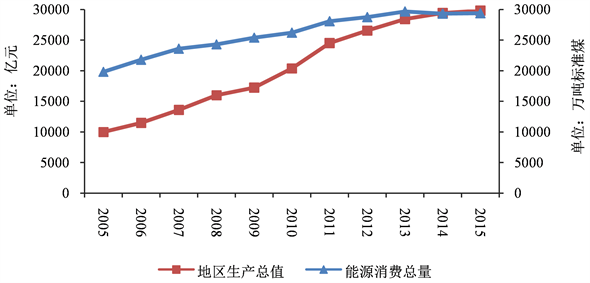Figure 1. Changes in gross domestic product and total energy consumption of Hebei province from 2005 to 2015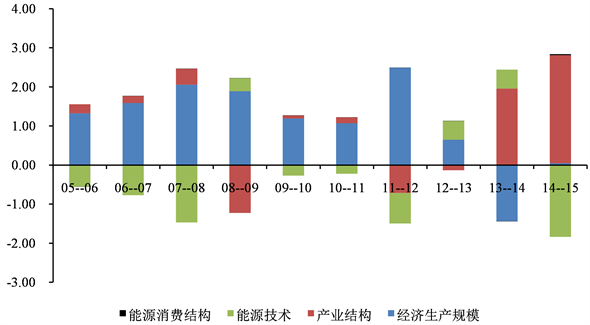Figure 2. The relative decomposition results of terminal energy consumption changes in Hebei Province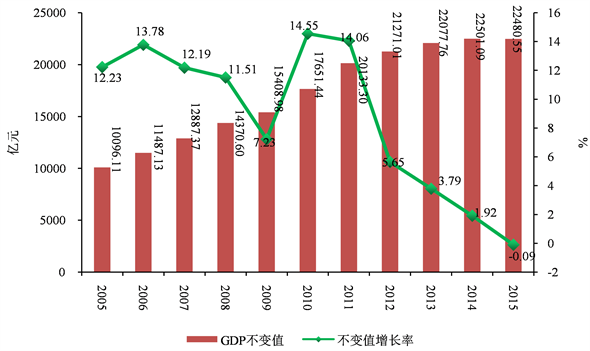Figure 3. Constant price economic production trend in Hebei Province

2013年之前，经济生产规模是河北省终端能源消费加大的决定性因素，在国家“三二一”产业发展的潮流下，诸如河北钢铁、河北石化等河北省支柱型产业逐渐失去了市场，而电子信息、现代物流、旅游等新兴第三产业还没有形成大规模生产，河北省就是处于这种“青黄不接”的时代，于是造成了经济发展不景气，随着产能过剩企业所占市场的份额减少，经济生产规模效应因此逐渐减弱，也就有了能源消费减少的现象。

3.2. 产业结构效应

3.3. 能源技术效应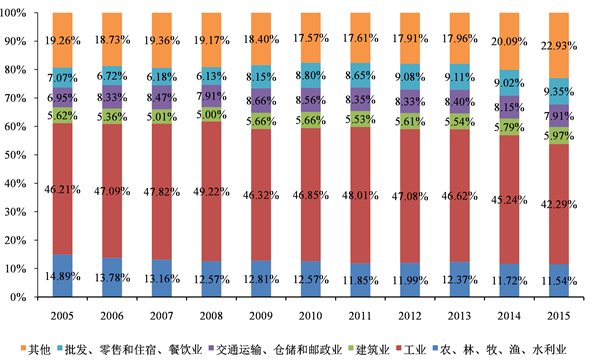Figure 4. Industrial sector structure in Hebei Province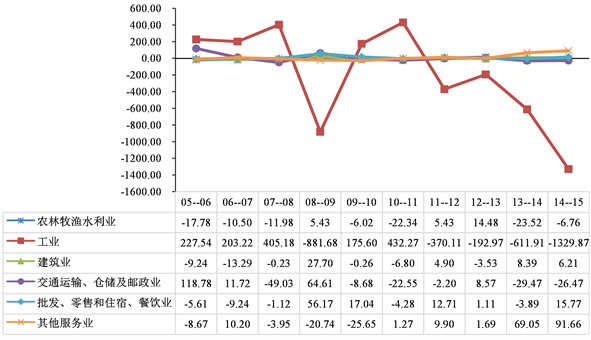Figure 5. The driving effect of industrial structure effect of each department (10,000 tons of standard coal)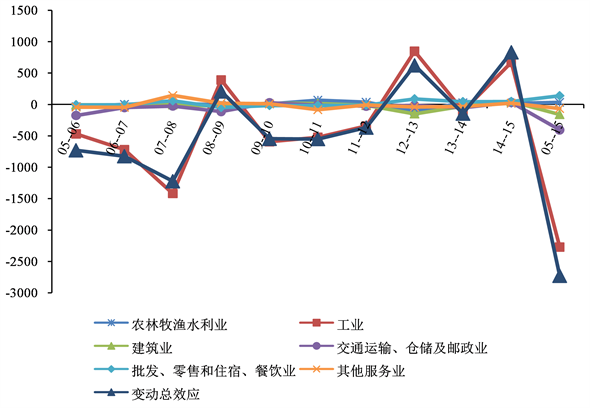Figure 6. The driving effect of energy technology effect of each department (10,000 tons of standard coal)

3.4. 能源结构效应

4. 政策性意见

4.1. 把握新常态：转换经济发展模式，优化产业结构调整，促进动力转化

4.2. 提高能源利用率，降低能源消耗

4.3. 调整能源消费结构，加大对优质能源的利用

 Jena, P. (2011) A Study of Changing Patterns of Energy Consumption and Energy Efficiency in the Indian Manufac-turing Sector. Institute for Environmental Economics and World Trade, University of Hannover, Hannover.

 Ang, B.W. (2005) The LMDI Approach to Decomposition Analysis: A Practical Guide. Energy Policy, 33, 867-871.
https://doi.org/10.1016/j.enpol.2003.10.010

 Hammond, G.P. and Norman, J.B. (2012) Decomposition Analysis of Energy-Related Carbon Emissions from UK Manufacturing. Energy, 41, 220 -227.
https://doi.org/10.1016/j.energy.2011.06.035

 Freitas, L.C. and Kaneko, S. (2011) Decomposition of CO2 Emissions Change from Energy Consumption in Brazil: Challenges and Policy Implications. Energy Policy, 39, 1495-1504.
https://doi.org/10.1016/j.enpol.2010.12.023

 郭朝先. 中国碳排放因素分解: 基于LMDI分解技术[J]. 中国人口•资源与环境, 2010, 20(12): 4-9.

 傅小里, 康侍民. 我国能源消费量影响因素分解及趋势分析[J]. 标准与检测, 2012, 40(260): 68-75.

 Ma, C.B. and Stern, D.I. (2008) China’s Changing Energy Intensity Trend: A Decomposition Analysis. Energy Economics, 30, 1037-1053.
https://doi.org/10.1016/j.eneco.2007.05.005

 马萍. 中国能源消耗强度影响因素分解分析[D]: [硕士学位论文]. 沈阳: 东北大学, 2009.

 Ma, C.B. (2010) Account for Sector Heterogeneity in China’s Energy Consump-tion: Sector Price Indices vs. GDP Deflator. Energy Economics, 32, 24-29.
https://doi.org/10.1016/j.eneco.2009.03.007

 李廉水, 周勇. 技术进步能提高能源效率吗?——基于中国工业部门的实证检验[J]. 管理世界, 2006(10): 82-89.

 郑义, 徐康宁. 中国能源强度不断下降的驱动因素——基于对数均值迪氏分解法(LMDI)的研究[J]. 经济管理, 2012, 34(2): 11-21.

 河北省发展和改革委员会. 河北省国民经济和社会发展第十三个五年规划纲要[Z], 2016.

 国家能源局. 能源技术创新“十三五”规划[Z]. 北京: 国家能源局, 2015.

Top